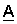# Phase Coordinates Method: Break Model

During operation of electric power systems, not only short-circuit modes can occur, but also breaks. The phase coordinate method allows you to simulate wire breakage.

In fig. 1 shows a circuit replacement circuit. At the point of wire breakage, a large active resistance is set (R = 1010 Ohms).Fig. 1. Break equivalent circuit

In the phase coordinate method, the break is described by the direct transfer matrix,

where.

Cliff modeling is performed using resistances,,. To simulate a break, for example, in phase A, the resistance is set equal to 1010 Ohms. Similarly model faults in other phases.

The attached file shows the calculation of the break matrixin Matlab: файл A_break.m

### Оставить комментарий

This site uses Akismet to reduce spam. Learn how your comment data is processed.

Подписаться
Уведомление о